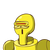# Find the equation of the straight line which passes through the point ( 3, -4) and having slope 3/2 *a) 3x – 4y –17 =

Find the equation of the straight line which passes through the point ( 3, -4) and having slope 3/2 *

a) 3x – 4y –17 = 0

b) 3x – 2y –17 = 0

c) 2x – 3y –17 = 0

d) 2x – 3y +17 = 0​

### 1 thought on “Find the equation of the straight line which passes through the point ( 3, -4) and having slope 3/2 *<br /><br />a) 3x – 4y –17 =”

1.